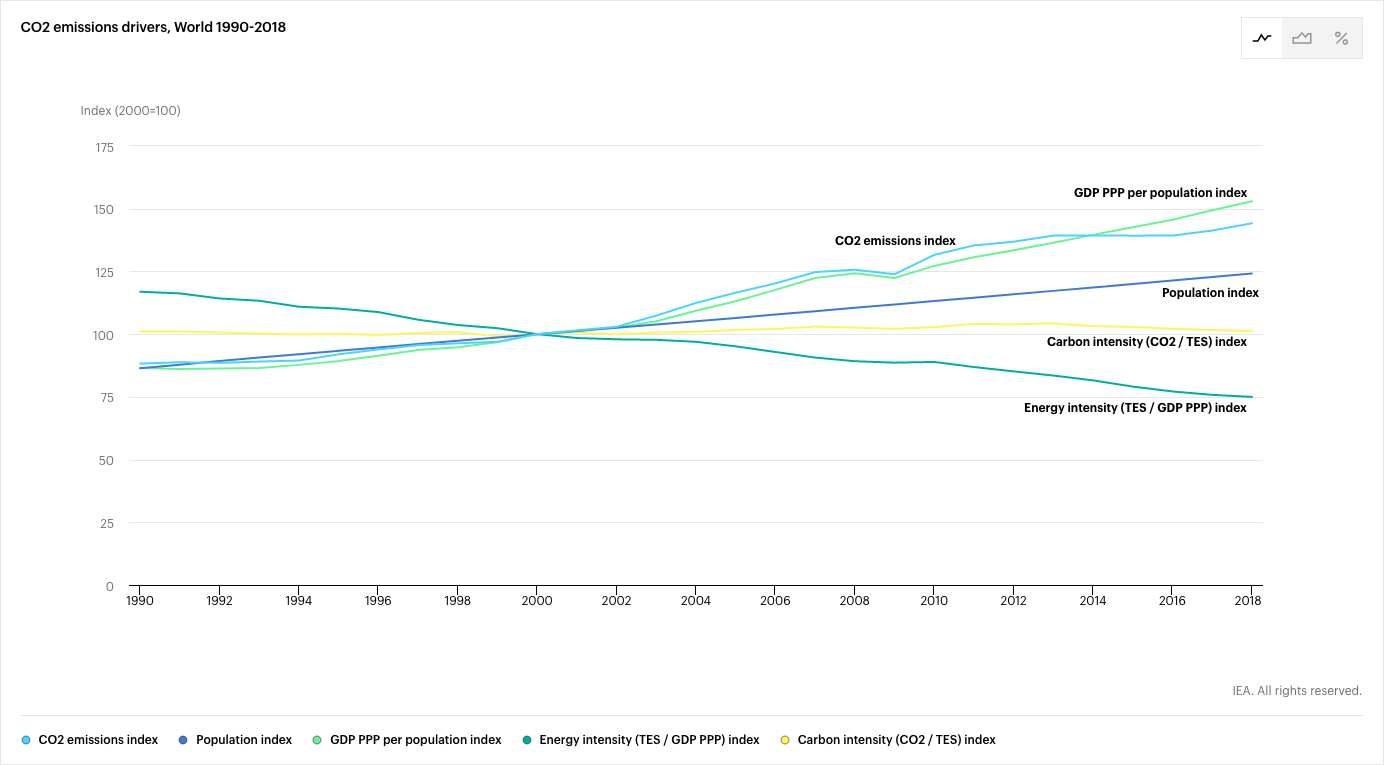# Have you met Kaya?

A few days ago I watched another video of Jean Marc Jancovici speaking at the MIT media lab. I can't say how many presentations of him I have seen in front of different audiences, but for once it was in English so it is appropriate to share it here. If you have never seen him talk, it is certainly worth two hours of your time.

## Tell me Kaya, what do you do?

A recurring theme of Jancovici's presentations is the Kaya identity:

\rm{CO_2} = \frac{\rm{CO_2}}{\rm{Energy}} \frac{\rm{Energy}}{\rm{GDP}} \frac{\rm{GDP}}{\rm{Population}} {Population}

Where

• \rm{CO_2} is the amount of \rm{CO_2} emissions.
• \rm{Energy} is the quantity of energy consumed.
• \rm{GDP} is the gross domestic product.
• \rm{Population} is the size of the population you consider (for instance the population of a country or the global population).

The identity is trivial as all the terms on the right side of the equation cancel to leave \rm{CO_2}=CO_2. What makes it interesting is that it ties the \rm{CO_2} emissions to the size of the population together with the following three factors:

• \frac{\rm{CO_2}}{\rm{Energy}} is the carbon footprint of the energy. It represents how much \rm{CO_2} (usually counted in tons of carbon or tons of \rm{CO_2}) is put in the atmosphere to produce one unit of energy.
• \frac{\rm{Energy}}{\rm{GDP}} is the energy intensity of the gross domestic product. It represents how much energy is needed to produce a given amount of money.
• \frac{\rm{GDP}}{\rm{Population}} is the gross domestic product per capita. It represents how much money each inhabitant produces on average.

Thus the Kaya identity proposes four ways to reduce the carbon footprint linked to the energy consumption of a given population:

1. To reduce the carbon footprint of the energy. This is why we often seek to replace carbon intensive power plants, like coal plants, by nuclear reactors or renewables (hydroelectric power plants, wind, solar, ...).
2. To reduce the energy intensity of the GDP. This corresponds to technological advances and optimizations: producing more with less energy.
3. To reduce the GDP per capita. Also called degrowth, it means (willingly or not) producing less per capita.
4. To reduce the population, all things being equal.

A big part of the discussions addressing climate change is: can technological solutions (1. and 2.) allow for a sufficient decrease of the global \rm{CO_2} emissions in a world where the GDP per capita (3.) and the population (4.) keep growing?The website of the International Energy Agency offers a really cool visualizer of the Kaya identity. It shows the evolution of the \rm{CO_2} emissions alongside the four parameters from the right side of the identity. The different parameters are shown as indexes meaning that they are normalized to their respective value in 2000. On the example graph shown above we see that \rm{CO_2} emissions worldwide have increased by 44% between 2000 and 2018, driven mainly by a growing population and a growing GDP per capita. The global carbon footprint of the energy is remarkably flat over the displayed period, while the energy intensity of the GDP has improved by 25% between 2000 and 2018. I invite you to click on the link above and play with the visualizer, where you can look at the Kaya identity for your own country.